Next: The Rigid Rotor Up: Some Analytically Soluble Problems Previous: The Particle in a

## The Harmonic Oscillator

Now consider a particle subject to a restoring force F = -kx, as might arise for a mass-spring system obeying Hooke's Law. The potential is then
 V(x) =
(118) =
If we choose the energy scale such that V0 = 0 then V(x) = (1/2)kx2. This potential is also appropriate for describing the interaction of two masses connected by an ideal spring. In this case, we let x be the distance between the masses, and for the mass m we substitute the reduced mass. Thus the harmonic oscillator is the simplest model for the vibrational motion of the atoms in a diatomic molecule, if we consider the two atoms as point masses and the bond between them as a spring. The one-dimensional Schrödinger equation becomes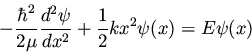(119)

After some effort, the eigenfunctions are
(120)

where Hn is the Hermite polynomial of degree n, and
and Nn are defined by(121)

The eigenvalues are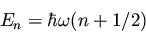(122)

with
.Next: The Rigid Rotor Up: Some Analytically Soluble Problems Previous: The Particle in a